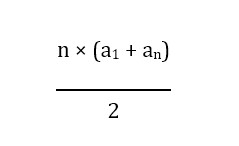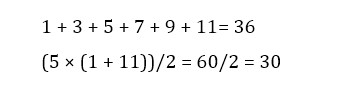# Arithmetic Sequence Calculator

an
=

## Arithmetic Sequence Calculator

Our online arithmetic calculator is the best calculator to evaluate the number sequence, generated by inserting a constant value. It may be used to locate any sequence property such as the common difference, the first term, the number of the first n terms, or nth term. You may actually delve into it or see how it functions. Arithmetic sequence calculator is also known as an arithmetic series calculator or just sequence calculator.

We will illustrate how to describe the arithmetic series, provide you the formula for an arithmetic sequence, and describe the calculator's sequence equation. We will discuss the applications of sequences, differences between arithmetical and geometrical sequences, difference between sequence and series, and many more.

## What is the Sequence?

A sequence is an organized set of objects in mathematics. A number sequence is, therefore, an organized set of numbers following a specific pattern. The length of a sequence is calculated by total number of terms in a sequence. Each term in a sequence referred to the each element in the sequence. The length of a sequence could be finite of infinite.

In a certain sequence, the sequence order is important, and the same terms can appear several times, depending on the sequence. There are many types of sequences, including Fibonacci sequences, arithmetical sequences, and geometric sequences are among the most popular.

## Applications of Sequences

Sequences in various mathematical disciplines have many applications because of their convergence properties. A series is converging when the sequence reaches certain limits, while a non-converging sequence is divergent. Sequences are used for the analysis of functions, spaces, and other mathematics concepts.

These are especially useful as a framework for a sequence commonly used in differential equations and the research area of mathematics. There are several ways to identify sequences, one of which is just to list the sequence if the sequence's pattern is easily recognizable.

Indexing is usually the preferred notation in cases with more complex patterns. Indexing include the development of a general form which allows the nth term of a series to be determined according to n function.

## What is Arithmetic Sequence?

An arithmetic sequence is a sequential representation of numbers in which the difference is always the same in each term. This difference can be positive or negative, and depending on the sign will lead to a positive or negative infinity sequence. The basic structure of an arithmetic series can be written:

an = a1 + f × (n-1)

Where an refers to the nth term in the sequence

an = am + f × (n-m)

a1 is the first term

a1, a1 + f, a1 + 2f

f is a common difference.

The common difference f is 2 in this sequence. Using the equation above to calculate the 6th term:

a6 = a1 + f × (n-1)

a6 = 1 + 2 × (6-1)

a6 = 1 + 10 = 11

Based on the list of sequences, the 6th term, a6, found using the equation corresponds as expected to the list of sequences. It is also always useful and easy to use the following formula and the previous formula to measure an arithmetic sequence description in order to find anThe sum of the arithmetic sequence is shown using the 6th term using and same number sequence.## Arithmetic sequence formula

Suppose you want the 28th term of any of the sequences mentioned above. It would be boring and time-consuming to write down the first 28 terms. But you probably realized you don't have to write all of them. Let us make this point simpler to make the equation of arithmetic sequence in general. This formula will be used for any nᵗʰ term of the sequence.

a = a + (n-1) d

In this equation, a₁ is the first term of the sequence, a is the nᵗʰ term of the sequence, d is a common difference.

For all common differences, whether positive, negative or zero, this arithmetic sequence formula applies for all cases. Of course, any single term is equivalent to each other in the case of a zero difference, rendering any computation pointless.

## Some Examples of Arithmetic sequence

Let’s go through some of the common examples of arithmetic sequence.

2, 4, 6, 8, 10, 12, 14, 16…

5, 2, -1, -4, -7, -10, -13, -16...

60, 60.1, 60.2, 60.3, 60.4, 60.5...

On the basis of these arithmetic sequence examples, you can see that the common difference is not a natural number. It is not essential to be a positive number, and it could also be a fraction in some cases.

It is called an increasing sequence if the common difference of an arithmetic series is positive. It will be called a decreasing sequence if the common difference is negative. If the common difference is zero, it will be a monotone sequence.

## Sequence vs. Series

The sum of the sequence called the arithmetic series is also calculated by our arithmetic sequence calculator.

Suppose there is an arithmetic sequence: 2, 4, 6, 8, 10, 12, 14, 16, 18. All of the terms in the sequence can be added manually, but that is not essential. We can add them in a more structured manner. Just add the first and the last term, then second and second the last term to each other.

2 + 18 = 20

4 + 16 = 20

6 + 14 = 20

The result of the addition of every pair is equivalent to 20 and remains constant. To calculate the sum of all terms in a sequence, there is no need to add all the terms individually. It will take time and effort. Just add the first and the last term of the sequence and multiply the result with the total number of terms in a sequence divided by two (n/2).

To represent it mathematically, it could be written as:

S = n/2 * (a + a)

After putting the equation of arithmetic sequence for nth term, it would become,

S = n/2 * [a + a + (n-1) d]

After further simplifying,

S = n/2 * [2a + (n-1) d]

Sum of an arithmetic sequence can be calculated by using this formula.

## Difference between Arithmetic and Geometric sequences

There are also other types of sequences except for arithmetic sequences. Geometric and Fibonacci sequences are more popular than others. Some sequences have no common difference, such as 2, 4, 6, 18, 34…, it is called a geometric sequence.

You may be wondering about the difference between geometric and arithmetic sequences. The main difference between arithmetic and geometric sequence is that the common difference is used to make the next terms in the arithmetic sequence while in a geometric sequence, the common ratio is used.

The digital universe is an interesting example of a geometrical sequence. The number of digital data doubles per two years. The data amounts in a geometric series can be written with a standard ratio of two.

## How to find Arithmetic Sequence using our recursive sequence calculator?

Our arithmetic sequence calculator is also known as a recursive sequence calculator. It has a very simple interface, and it is very easy to use. Most of the arithmetic sequence calculators lack the simplicity in user interface resulting in complications which user don’t understand when giving the input. We have made the input and output of this calculator very easy and smooth.

To use this calculator, enter the values in the given input boxes. First of all, enter the nth term. It means you have to enter, for example, a3, a5, a6, etc., and its corresponding value in the next text box. Enter the common difference of the sequence in the text box labeled as “Common Difference.” Finally, enter the total number of terms in the sequence, which is simply denoted by n. Press the “Calculate” button to see the results.

It will provide the results instantly. It will give you the results in complete detail such as nth term, the sum of the nth terms in your sequence, and then the complete sequence itself. It also shows in the results that how it calculated the sequence for your query. It shows the step by step solution and formulas applied to calculate the sequence and other relevant things.

You will not just see the results in written form when using our arithmetic sequence calculator. We offer a graphical representation of the result in our arithmetic sequence graph. You can see the graph drawn by our calculator to represent the arithmetic sequence after the result. If you have difficulty in understanding the results, you can simply use the graph to understand the arithmetic sequence populated by the calculator against your given input. It is a great feature for students and teachers because sometimes, textual representation becomes very boring, and graphical representation is a great way to absorb information by just looking at it.

#### Need Custom Calculator?

With Over Online Tools, eCalculator.co Helping Millions of Students, Teachers, Businessmen & Nutritionists Every Month.